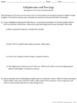# Pythagorean Theorem Discovery WorksheetsSubject
Resource Type
File Type

PDF

(3 MB|14 pages)
Standards
Also included in:
1. This eighth grade math bundle contains discovery worksheets, card games, task cards, card sorts, stations, scavenger hunts, and more! All activities are aligned to the eighth grade math Common Core State Standards. This is a subset of my 8th Grade Math MEGA (Growing) Bundle. It is offered for tea
\$203.75
\$140.00
Save \$63.75
2. This eighth grade math MEGA (growing) bundle will eventually be a full curriculum. It currently contains all discovery worksheets, card games, task cards, card sorts, stations, scavenger hunts, and more! All activities are aligned to the eighth grade math Common Core State Standards.Check out the
\$480.25
\$300.00
Save \$180.25
• Product Description
• StandardsNEW
In these three Common Core-aligned discovery worksheets, students will be able to use the Pythagorean Theorem to solve for a missing side of a right triangle, solve word problems involving right triangles, determine the distance between two points using the Pythagorean Theorem, and test for right triangles using the converse of the Pythagorean Theorem.

Included:
-3 two-page worksheets (with answer keys)
-Teacher's Guide with CCSS, objectives, materials, and procedure

Worksheet #1: Using the Pythagorean Theorem
Students will learn how to solve for a missing side of a right triangle using the Pythagorean Theorem. They will reflect on how they know which side is the hypotenuse. They will also use the Pythagorean Theorem to find missing side lengths of two-dimensional and three-dimensional geometric figures, and students will then use that information to determine measurement such as perimeter and surface area.

Worksheet #2: Pythagorean Theorem in the Real World
In this activity, students will understand how to draw pictorial representations of right triangles represented in word problems to help them write and solve equations using the Pythagorean Theorem. Then students will be able to determine the distance between two points using the Pythagorean Theorem by practicing with a basic problem and a multi-part word problem. Students may need to review the equation d=rt.

Worksheet #3: Pythagorean Theorem and its Converse
Students will identify if a set of three side lengths could form a right triangle by using the Pythagorean Theorem, and they will reflect on how they know which side length must be the hypotenuse. Then all previously mentioned ideas are reviewed with a practice problems.

This product is part of the Discovery-Based Worksheet Series. Discovery-Based Worksheets have been specially designed to engage students in learning that moves beyond traditional skills practice. Students will develop a deeper understanding of the big idea and will make connections between concepts. These worksheets make a great introduction to a new topic or summary at the end of a lesson or unit. Try one today!

You might also like:
Pythagorean Theorem Differentiated Notes and Practice
Converse of Pythagorean Theorem Differentiated Notes and Practice
Pythagorean Theorem Stations
Proving Pythagoras Right: Proving the Pythagorean Theorem
Thanksgiving: Applying the Pythagorean Theorem

Teach 8th grade math? Consider this incredible deal: 8th Grade Math MEGA (Growing) Bundle

This resource is also included in the 8th Grade Math Discovery and Hands-On Activity Bundle.

**************************************************************************
☺ Earn TpT credits to use toward future purchases by providing feedback for paid products. (Feedback on free products is also greatly appreciated!)
☺ Follow me to receive notifications when new products are posted. This will help you secure the 50% discount available for the first 48 hours. You will also receive occasional messages alerting you to sales, promotions, and other exciting news.
☺ Don’t quite see what you are looking for? Leave me a note in the “Ask a Question” tab. I would be happy to create a product to meet the needs of you and your students.

The purchase of this product entitles a single user to reproduce the resource for classroom use only. A multiple license can be purchased if you wish to share with other teachers. The product is for educational use only. The product may not be used for commercial purposes or resold in any form. It cannot be uploaded to the Internet, including school websites.
© Free to Discover (Amanda Nix)

Apply the Pythagorean Theorem to find the distance between two points in a coordinate system.
Apply the Pythagorean Theorem to determine unknown side lengths in right triangles in real-world and mathematical problems in two and three dimensions.
Total Pages
14 pages
Included
Teaching Duration
3 days
Report this Resource to TpT
Reported resources will be reviewed by our team. Report this resource to let us know if this resource violates TpT’s content guidelines.